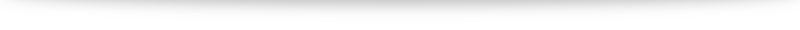# Integration techniques

1. Equations and inequalities
2. Curves and transformations
3. Functions
4. Arithmetic and geometric progressions
5. Series and the sigma notation
6. Differentiation
7. Maclaurin series and binomial expansion
8. Integration techniques
9. Definite integrals
10. Differential equations
11. Vectors
12. Complex numbers

Next up: randomly generated question on integration to test your concepts. Then vectors, which will likely take a whole chunk of time and effort. Let’s work hard together!# Equations and inequalities

The first set of theory discussion and worked examples are up! I welcome feedback on what was helpful for you and what could be improved, both on the mathematics side as well as the website usability side. I intend to add one or two more randomly generated questions for this topic, and perhaps add some links between the theory discussion, the randomly generated question and the worksheet for the topic. Otherwise I think the discussion should cover the basics of what you will need for the exams.# Hello world!

$\mu \alpha \theta \eta \mu \alpha \tau \iota \kappa \alpha$. Welcome to $\mathbf{a} \cdot \mathbf{b}$.